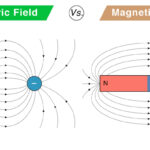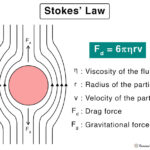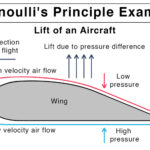Home / Physics / Rolling Friction

# Rolling Friction

## What is Rolling Friction

When an object rolls over the surface, it experiences resistance to its motion. This resistive force is a type of kinetic friction and known as rolling friction. The point at which the object is in contact with the surface is critical in understanding rolling friction. At this point, both the object and the surface deform due to factors like elasticity, weight, and roughness. The cause of rolling friction is this deformation. It is also known as rolling resistance. A special form of rolling friction is called traction. A typical example is the friction between the tire and the ground.

## Rolling Friction Examples

The rolling friction can be observed in daily life. Here are some examples.

• When a soccer ball is kicked, it stops after rolling on the ground.
• When a golf ball is hit, it stops on the green after rolling.
• A car will come to a stop after rolling downhill and reaching a flat road.
• A skateboard moving on wheels will come to a stop after some time.
• A roller coaster slows down its ride towards the end due to a flat strip of track.

## Laws of Rolling Friction

Here are some laws of rolling friction acting on a round object rolling on a surface

• Directly proportional to the normal load
• Inversely proportional to the radius of curvature
• Decreases as the surface roughness is reduced, i.e., less for smoother surfaces

## Rolling Friction Equation

Consider an object of mass m rolling over a horizontal surface. Its weight is given by mg, where g is the acceleration due to gravity. According to Newton’s laws of motion, the object’s weight will be balanced by the normal force FL.

FL = mg

Also, from laws of friction, the rolling frictional force FR is proportional to the normal load.

FR FL

Or, FR = μR FL

Or, FR = μR mg

The constant of proportionality is called the coefficient of rolling friction. It takes a constant value depending on the nature and material of the surfaces. It is unitless and dimensionless, whereas the unit of rolling friction is Newton or N. For example, the coefficient of rolling friction between the tire and road is 0.7 for dry road and 0.4 for the wet road.

## Rolling Without Slipping

Rolling is generally a combination of translation and rotation motion. When an object is rolling without slipping, the point in contact with the surface is always at rest instantaneously. Under such circumstances, we have the following equations.

v = wR

a = R

Where, v is the translational velocity, w is the angular velocity, a is the translational acceleration, is the angular acceleration, and R is the radius of the object.

The rolling friction force prevents the object from slipping. Its direction is the same as that of the translational velocity. If the object rotates clockwise on a flat surface, the friction will be directed towards the right. Similarly, if the object is rolling up a hill, the friction will be directed upwards. Also, since the point of contact is stationary, the work done by friction in pure rolling is zero.

Here are some advantages of rolling friction.

• Reduces the friction between an object and a surface, i.e., lower than sliding friction
• Allows an object to move smoothly over a surface
• Allows an object to roll up a hill
• Luggage fitted with wheels roll easily

Article was last reviewed on Thursday, November 26, 2020

### Related articlesElectric Field vs. Magnetic FieldHooke’s LawStokes’ LawBernoulli’s Principle and Equation